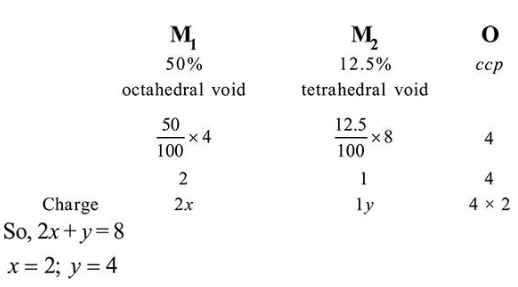# A crystal is made up of metal ions 'Question:

A crystal is made up of metal ions ' $\mathrm{M}_{1}$ ' and ' $\mathrm{M}_{2}$ ' and oxide ions. Oxide ions. form a $c c p$ lattice structure. The cation ' $\mathrm{M}_{1}$ ' occpies $50 \%$ of octahedral voids and the cation ' $\mathrm{M}_{2}$ ' occupies $12.5 \%$ of tetrahedral voids of oxide lattice. The oxidation numbers of ' $\mathrm{M}_{1}$ ' and ' $\mathrm{M}_{2}$ ' are, respectively:

1. $+2,+4$

2.  $+1,+3$

3. $+3,+1$

4.  $+4,+2$

Correct Option: 1

Solution: